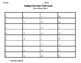Subject
Resource Type
File Type

PDF

(1 MB|19 pages)
Standards
• Product Description
• StandardsNEW
This file contains 32 task cards where students will add fractions with unlike denominators. These cards may be used in centers, as exit tickets, or even as a math scoot. I've also included 3 worksheets that can be used for classwork or homework. Answer keys are included.

Decompose a fraction into a sum of fractions with the same denominator in more than one way, recording each decomposition by an equation. Justify decompositions, e.g., by using a visual fraction model. Examples: 3/8 = 1/8 + 1/8 + 1/8; 3/8 = 1/8 + 2/8; 2 1/8 = 1 + 1 + 1/8 = 8/8 + 8/8 + 1/8.
Understand addition and subtraction of fractions as joining and separating parts referring to the same whole.
Understand a fraction 𝘢/𝘣 with 𝘢 > 1 as a sum of fractions 1/𝘣.
Total Pages
19 pages
Included
Teaching Duration
N/A
Report this Resource to TpT
Reported resources will be reviewed by our team. Report this resource to let us know if this resource violates TpT’s content guidelines.